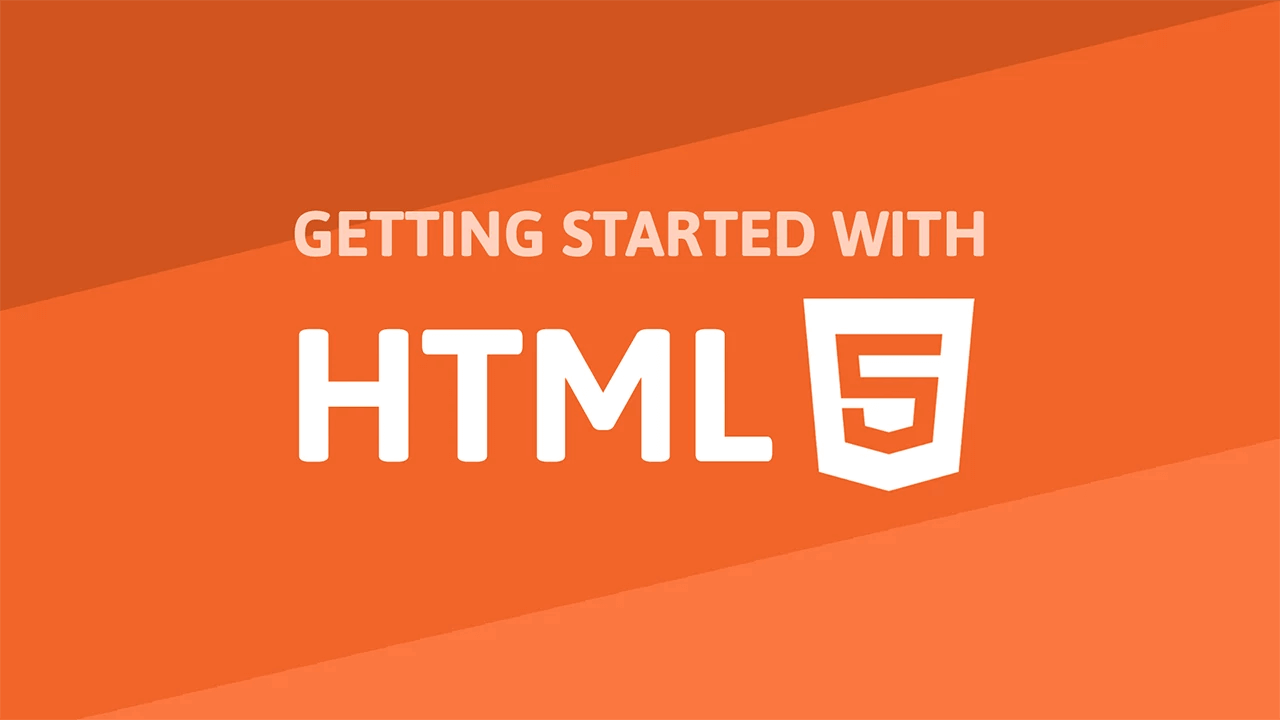# Displaying Data with TablesTables in HTML let you organize and arrange data into a set number of rows and columns of cells. They are very useful whenever you want to display tabular data. After all, that's what it was made for in the first place.

## Tables

To create your very first table, use the `table` tag. Tables use multiple tags to render properly, and they are as follows:

• table: Surrounds and defines the table's start and end.
• tr: Stands for a table row. It surrounds a row.
• th: Stands for a table header.
• td: Stands for a cell of table data.

To get a better idea of how tables work, here is the structure of a simple 3 by 3 table:

```	```
<!DOCTYPE html>
<html>
<title>Table</title>
<body>
<h1>I am a 3x3 table.</h1>
<table>
<tr>
</tr>
<tr>
<td>(1, 1)</td>
<td>(1, 2)</td>
<td>(1, 3)</td>
</tr>
<tr>
<td>(2, 1)</td>
<td>(2, 2)</td>
<td>(2, 3)</td>
</tr>
<tr>
<td>(3, 1)</td>
<td>(3, 2)</td>
<td>(3, 3)</td>
</tr>
</table>
</body>
</html>
```
```

### Row and Column span

There are two optional attributes you can give to your `td` tags, and they are `colspan` and `rowspan`.

You can make cells span either multiple rows or multiple columns. This is perhaps better illustrated with an example:

```	```
<table>
<tr>
</tr>
<tr>
<td>(1, 1)</td>
<td colspan="2">(1, 2) AND (1, 3)</td>
</tr>
<tr>
<td rowspan="2">(2, 1) AND (3, 1)</td>
<td>(2, 2)</td>
<td>(2, 3)</td>
</tr>
<tr>
<td>(3, 2)</td>
<td>(3, 3)</td>
</tr>
</table>
```
```

### Captions

Tables can have captions, which you can define by using the `caption` tag right after the `table`tag.

```	```
<table>
<caption>This is a table caption.</caption>
<tr>
</tr>
<tr>
<td>(1, 1)</td>
<td>(1, 2)</td>
</tr>
<tr>
<td>(2, 1)</td>
<td>(2, 2)</td>
</tr>
</table>
```
```

If your table is particularly large, or you just want a row to be rendered at the top or bottom of the table, you can utilize the `thead` (for table header) and `tfoot` (for table footer) tag. However, once you use these tags, you should then also use the `tbody` tag to define the body of the table.

```	```
<table>
<caption>This is a table caption.</caption>
<tr>
</tr>
<tbody>
<tr>
<td>(1, 1)</td>
<td>(1, 2)</td>
</tr>
</tbody>
<tfoot>
<tr>
<td>(2, 1)</td>
<td>(2, 2)</td>
</tr>
</tfoot>
</table>
```
```

That's pretty much it for tables. In our next lesson, we'll cover forms and how to get input from the user.